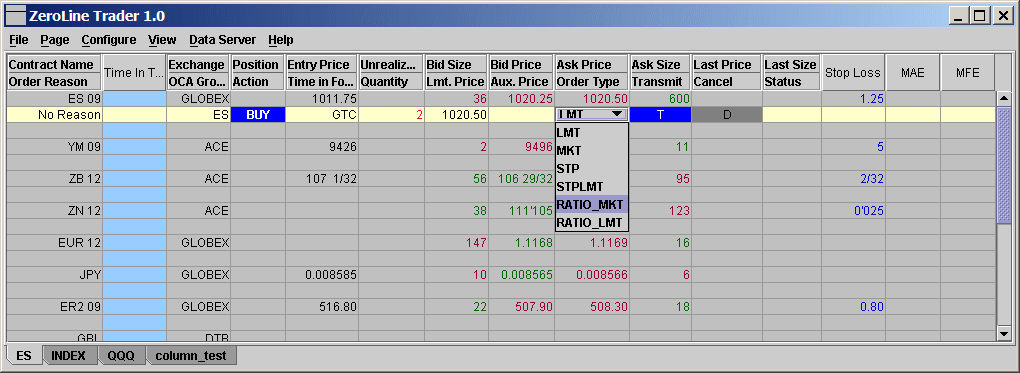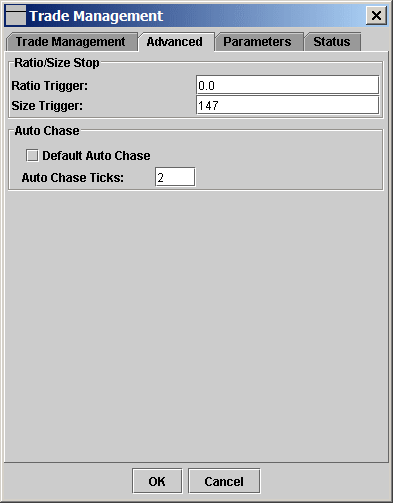## Ratio/Size Stop

There are two types of ratio/size stop: RATIO_MKT and RATIO_LMT. They can be used as stop loss order to close positions or as regular stop orders to establish new positions.

Use Ratio/Size Stop as Stop Order

To use the ratio stop as stop loss order, just select RATIO_MKT or RATIO_LMT in Trade Management dialog for the Stop Loss Order Type.Use Ratio/Size Stop as Entry Order

To use the ratio stop to establish new position, just select RATIO_MKT or RATIO_LMT in the Order Type combo box.Parameters

The ratio/size stop parameters are defined in the Trade Management dialog's Advanced tab.Ratio Trigger: The bid size/ask size ratio (for sell ratio stop) or ask size/bid size ratio (for buy ratio stop) has to be lower than this Ratio Trigger value to trigger the ratio stop order.

Size Trigger: The bid size (for sell ratio stop) or the ask size (for buy ratio stop) has to be below this value to trigger the ratio stop order.

Once either Ratio Trigger or Size Trigger is satisfied, the ratio stop will be triggered.

How Ratio Stops Work

Once the ratio stop order is triggered, if it is a RATIO_MKT, it will generate and transmit a MKT order to buy or sell; if it is a RATIO_LMT, it will generate and transmet a LMT order with the given limit price to buy or sell.

For buy ratio stop, once the contract's ask price is equal to the aux price of the ratio stop order, the program will check if the ratio of ask size/bid size is smaller than the ratio trigger, or if the ask size is smaller than the size trigger, if one of them is true, a limit order (for RATIO_LMT) or a market order (for RATIO_MKT) will be transmitted to TWS.

For sell ratio stop, once the contract's bid price is equal to the aux price of the ratio stop order, the program will check if the ratio  of bid size/ask size is smaller than the ratio trigger, or if the bid size is smaller than the size trigger, if one of them is true, a limit order (for RATIO_LMT) or a market order (for RATIO_MKT) will be transmitted to TWS.

The ratio stop orders reside in ZLT, if the connection between ZLT and TWS is lost, the ratio stop orders won't be able to transmit orders to TWS. So the ratio stop orders won't work during disconnection.

On the other hand, the ratio stop orders will not reveal your stop prices.

Here is an example of using ratio stop: Just assume you want to buy ES either to establish new long position or to close a short position. The current bid/ask price is 999.75/1000.00 and the bid size/ask size is 50/600. So there is 600 contracts sitting on the ask. Do you want to buy immediately, while the ask size/bid size ratio is 12?

Now if you use a RATIO_LMT order with aux price 1000.00 and limit price 1000.00 and the ratio trigger 10, this RATIO_LMT order will not be triggered (even it has traded at 1000.00) until the ask size/bid size ratio is less than 10. Once the ask size/bid size ratio is less than 10, for example the bid size/ask size becomes 50/400 after some one buy at ask for 200 contracts, a  buy limit order with 1000.00 as limit price will be sent.

Similarly, if you use RATIO_MKT with aux price 1000.00, once the ask size/bid size ratio is less than 10, a buy market order will be transmitted.

One benefit to use the ratio order as stop order is your stop will not be picked. In above example, your stop order at 1000.00 to close short position will not be transmitted until the sellers at the ask price has vanished/been taken out to a certain level. And your stop order is not visible.

One  risk is if the connection between ZT and TWS is lost, the stop loss order can not be transmitted. But this situation is possible but rare and you can reconnect to TWS if it happens. However, if the internet connection is down or the exchange is down, we can do nothing. The stop is not queued at the exchange.

Another risk is your order may not be executed. In above example, if the 600 contracts at ask is taken out by one trade, the bid/ask price immediately move to next level 1000.00/1000.25 and keeps moving up, then the lmt buy order at 1000.00 generated by the RATIO_LMT may or may not be filled. However, if you use RATIO_MKT, the market order will be filled at the market price which may be higher than 1000.00.

In summary, the RATIO_LMT or RATIO_MKT just use the ratio between bid size and ask size as a trigger to generate LMT order or MKT order. Note the trigger is always the ratio is less than the given value specified in Trade Management dialog. So for buy order, we compare ask size/bid size ratio but for sell order we compare bid size/ask size ratio.

The STPLMT and STP orders usually use the last price as trigger. If so, once one trade is at 1000.00, your stop order is converted to limit order or market order while the bid size/ask size is still at 50/599.

Backup Order

RATIO_LMT and RATIO_MKT orders are handled and triggered by ZLT on your local computer. In case the Internet connection between your computer and the broker is lost, it is impossible to transmit them to the broker or the exchange. So ZLT provides the backup order which has the order type that is either hosted by the broker's server or the exchange's server.